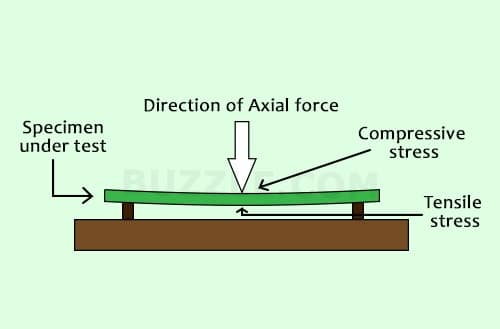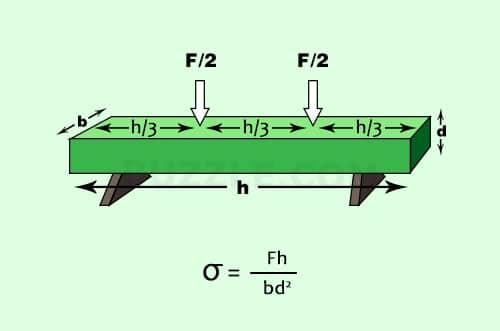Sorry, you do not have permission to ask a question, You must login to ask question. Become VIP Member

Do you need to remove the ads? Become VIP Member

Modulus of rupture is a measure of the tensile strength of concrete beams or slabs. Flexural strength identifies the amount of stress and force an unreinforced concrete slab, beam or other structure can withstand such that it resists any bending failures. Modulus of rupture is also known as flexural strength,bend strength or fracture strength.Fig 1: Flexural Stress in a loaded Beam.

## Standard Test Methods to Determine the Modulus of Rupture of a Beam

In order to test the flexural strength of a concrete beam, its span length should be at least three times the depth. The flexural strength is expressed as the modulus of rupture (MR) in psi (MPa). There are two standard test methods to determine the flexural strength of a concrete beam,

In this test method, the entire load is applied at the center of the beam’s span length. Here the flexural strength or modulus of rupture is higher than the modulus of rupture of the third point loading test. The maximum stress is present only at the center of the beam.In this test method, half the load is applied at each third of the beam’s span length. Here the flexural strength or modulus of rupture is lower than that of the modulus of rupture found in the center point loading test. In this test, the maximum stress is present over the center one-third portion of the beam.Flexural modulus of rupture is about 10% to 20% of the compressive strength depending on the type, size and volume of coarse aggregate used in a concrete beam. However, the best correlation for specific materials is obtained by laboratory tests for the given materials and mix design. The modulus of rupture determined by third point loading is lower than the modulus of rupture determined by center point loading, sometimes by as much as 15%.

## Calculation of Modulus of Rupture

The formula for modulus of rupture differs for different type of loading system,

### 1. For a rectangular sample under a load in a three-point bending setup

Equation 1:• F is the load (force) at the fracture point (N)
• L is the length of the support span
• b is width
• d is thickness

### 2. For a rectangular sample under a load in a four-point bending setup where the loading span is one-third of the support span

Equation 2:• F is the load (force) at the fracture point
• L is the length of the support (outer) span
• b is width
• d is thickness

### 3. For the 4 pt bend setup, if the loading span is 1/2 of the support span

Equation 3:### 4. If the loading span is neither 1/3 nor 1/2 the support span for the 4 pt bend setup

Equation 4:## Difference between Rupture and Tensile Strength

There is always the possibility of some local defects being present in any object. When an object is put under tensile stress it tends to ‘expand’. All the fibers or regions of an object experience an equal force. Any weak regions in such a case may give up and undergo deformation.Fig 4: Difference between Rupture and Tensile Strength

Flexural stress, on the other hand, exerts both tensile and compressive force upon an object. This results in unequal distribution of forces among the fibers of the objects. The ‘extreme fibers’, i.e., the fibers at the surface of the object tend to experience maximum forces. Therefore, they are most vulnerable to breakage or rupture.

## Importance of Modulus of Rupture

The calculation of modulus of rupture is considered crucial in structural mechanics,

1. It helps in designing structural elements like beams, cantilevers, shafts, etc.
2. Aids in the study of materials and their properties.
3. Provides a parameter for development of stronger constructional materials.
4. Flexural strength helps in judging the quality of structures being used for construction.
5. It is a tool to predict both resistance and durability of objects.

EDITOR